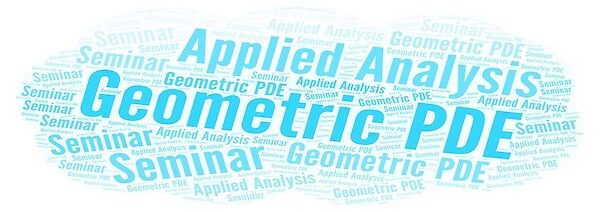# [Seminar] "Liouville theorem for V-harmonic maps under non-negative (m, V)-Ricci curvature for non-positive m" by Prof. Kazuhiro Kuwae### Date

Thursday, October 27, 2022 - 16:00

L4E01 or Zoom

### Description

Geometric PDE and Applied Analysis Seminar (October 27, 2022)

Title: Liouville theorem for $$V$$-harmonic maps under non-negative $$(m, V)$$-Ricci curvature for non-positive $$m$$

Speaker: Prof. Kauzhiro Kuwae (Fukouka University)

Abstract: This talk is based on a joint work with Xiangdong Li (CAS AMSS), Songzi Li (Renming University of China) and Yohei Sakurai (Saitama University). We consider a generalization of bounded Liouville property for $$V$$-harmonic maps under non-negative Ricci curvature in terms of $$m$$-Bakry-Émery Ricci tensor for non-positive $$m$$. This condition is quite weaker than the non-negativity of  usual $$m$$-Bakry-Émery Ricci curvature for which $$m$$ is greater than the dimension $$n$$ of the source Riemannian manifold. We establish a Liouville type theorem of $$V$$-harmonic maps into Hadamard manifolds having a growth condition which depends on the shape of $$V$$-Laplacian comparison theorem under such non-negative $$m$$-Bakry-Émery Ricci curvature. We prove the result by use of stochastic analysis. Of course, one can prove the result by purely geometric analysis.